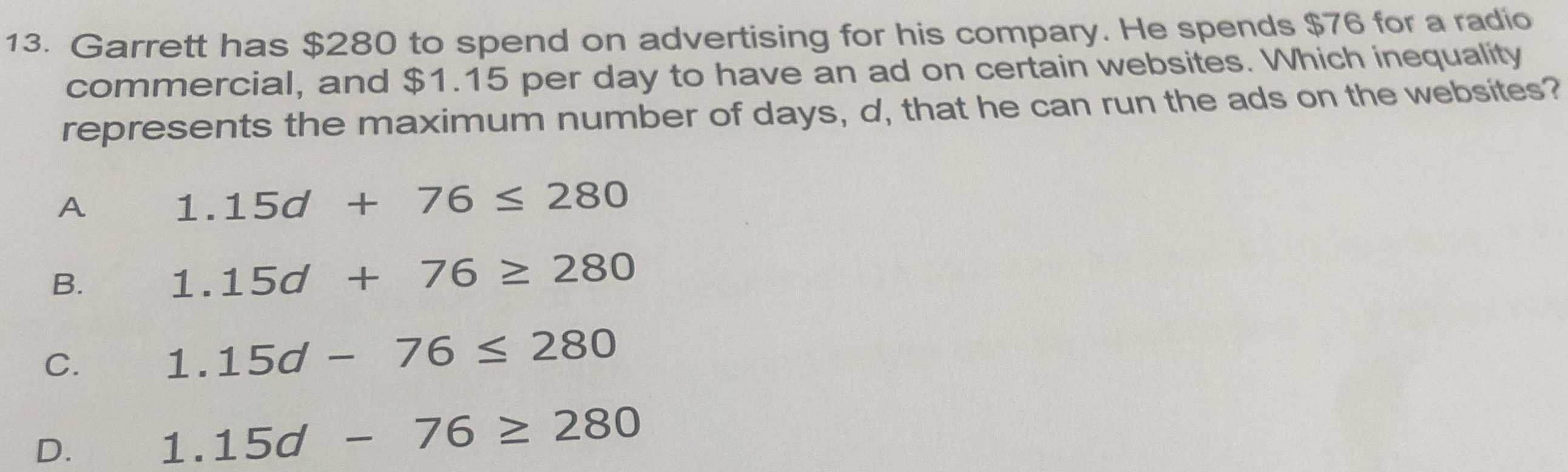### Still have math questions?

Arithmetic
Question13. Garrett has $$\ 280$$ to spend on advertising for his compary. He spends $$\ 76$$ for a radio commercial, and $$\ 1.15$$ per day to have an ad on certain websites. Which inequality represents the maximum number of days, $$d$$ , that he can run the ads on the websites? A $$1.15 d + 76 \leq 280$$

B. $$1.15 d + 76 \geq 280$$

C. $$1.15 d - 76 \leq 280$$

D. $$1.15 d - 76 \geq 280$$